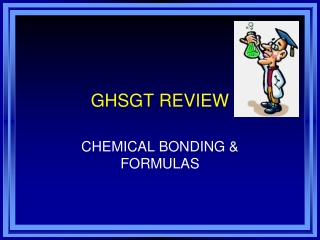DownloadDownload PresentationGHSGT REVIEW

# GHSGT REVIEW

Télécharger la présentation## GHSGT REVIEW

- - - - - - - - - - - - - - - - - - - - - - - - - - - E N D - - - - - - - - - - - - - - - - - - - - - - - - - - -
##### Presentation Transcript

1. GHSGT REVIEW CHEMICAL BONDING & FORMULAS

2. What is a chemical bond? • The attractive force that holds atoms or ions together in a compound.

3. What is the goal when atoms bond? • To obtain a full outer energy level

4. What is the octet rule? • An atom will gain, lose or share electrons to get 8 electrons on the outermost energy level • Full outer energy level = stable atom

5. What are two types of bonds that can occur between atoms? • Ionic • Covalent

6. What is an ionic bond? • A bond formed by atoms that have gained or lost electrons

7. Example: F p+ 9F1- p+ 9 no 10no 10 e- 9e- 10 Na p+ 11Na1+ p+ 11 no 12no 12 e- 11e- 10

8. The ionic compound formed is NaF (sodium fluoride) • Na+ was attracted to F-

9. SODIUM CHLORIDE

10. What is a covalent bond? • A bond formed when atoms share one or more pairs of electrons

11. What happens to the electrons when a covalent bond forms? • Electrons in the outermost energy level are shared between the two atoms • Shown using an electron dot diagrams.

12. COVALENT BOND

13. How can the type of bond between two atoms be predicted? • Metal and nonmetal = ionic bond • Nonmetal and nonmetal = covalent bond Predict the following: Mg and F = ionic O and Cl = covalent Zn and Zn = metallic

14. What is a polyatomic ion? • An ion made of two or more atoms that are covalently bonded and that act like a single ion; usually forms ionic bonds with other atoms

15. Compound Names and Formulas

16. What is an oxidation number? • The number of electrons an atom needs to gain, lose, or share when it forms a chemical bond • Pattern of oxidation numbers: +1 +2 +3 + or -4 -3 -2 -1 0 Ex: Na = +1 Cl = -1 Mg = +2 O = -2

17. How can oxidation numbers be used to write chemical formulas for ionic compounds? • Write the symbols of the atoms • Write the correct oxidation numbers to the right of each symbol • If the oxidation numbers do not add up to zero, write the oxidation number of each atoms as a subscript of the opposite symbol (the symbol of the atom with the positive oxidation number is written 1st in the formula)

18. This is called the “criss-cross” method • Ex: Lithium and fluorine Li1+ F1- = LiF Magnesium and chlorine Mg2+ Cl1- = MgCl2

19. If the compound is covalent, what rules are followed to write the formula? • Prefixes will be used in the name of the formula so just write the symbols of the elements and use the prefix (di, tri, etc.) as the subscript. DO NOT USE OXIDATION NUMBERS TO WRITE THE FORMULAS FOR COVALENT COMPOUNDS!!! DO NOT USE THE “CRISS-CROSS” METHOD!!!!

20. Ex: carbon dioxide = CO2 carbon tetrachloride = CCl4

21. What are the common prefixes? 1 = mono- 2 = di- 3 = tri- 4 = tetra- 5 = penta- 6 = hexa- 7 = hepta- 8 = octa- 9 = nona- 10 = deca-

22. What are the rules for naming compounds? • If the compound is a metal and a nonmetal, change the ending of the nonmetal to –ide • Ex: NaBr = sodium bromide AlCl3 = aluminum chloride

23. If the compound has a polyatomic ion, use the name of the polyatomic ion in the name of the compound. • Ex: AgNO3 = silver nitrate Na2CO3 = sodium carbonate

24. If the compound is 2 nonmetals, use the prefixes such as di, tri, etc. of the subscript in the name - also change the ending to –ide • Ex: SiO2 = silicon dioxide NF3 = nitrogen trifluoride